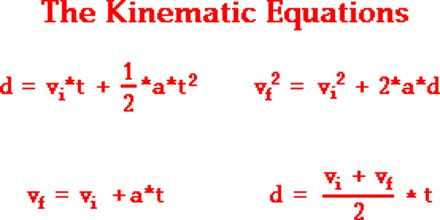Physics

# Kinematic EquationsMajor objective of this lecture is to present on Kinematic Equations. Here describe the definitions of position, distance, displacement, speed, velocity and acceleration and distinguish whether these are scalars or vectors. Here also explain the equations of motion involving distance/displacement, speed/velocity, acceleration and time in calculations and in interpreting experimental results. Finally explain Calculus formulas and Accelerated Motion.